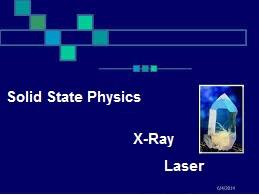# Online Preliminary Examination-may,2020 For B.Sc.-IV Semester,Organized By Department Of Physics,Sgmm Hingna,Dist-nagpur

25 Questions | Total Attempts: 68SettingsCreate your own QuizB. Sc. Sem-IV Instructions for examination: The Quiz is based on the R. T. M. Nagpur University Syllabus of B. Sc. (Physics). B. Sc. Second Year (Semester IV) Course-PHYSICS - Paper-I (401) (Solid state physics, X-ray and Laser) The Quiz consist of total 25 multiple choice questions. Each question carry TWO marks All Questions are Compulsory. No negative marking Has a time limit of 120 minutes Has a pass mark of 50% You can download certificate after finishing the quiz.

• 1.
Miller indices for plane having intercept 3a, 3b, 2c with respective X, Y, Z axes are
• A.

(323)

• B.

(332)

• C.

(223)

• D.

(333)

• 2.
Number of atoms per unit cell for FCC structure is
• A.

0

• B.

1

• C.

2

• D.

4

• 3.
Coordination number for an ideal BCC metallic crystal is:
• A.

4

• B.

6

• C.

8

• D.

12

• 4.
The smallest portion of the lattice is known as __________
• A.

Bravais crystal

• B.

Unit cell

• C.

Lattice point

• D.

Lattice structure

• 5.
Which of the following axis system is being satisfied by cubic crystal system?
• A.

A = b = c, α = β = γ = 900

• B.

A = b ≠ c, α = β = γ = 900

• C.

A = b = c, α ≠ β = ϒ = 900

• D.

A ≠ b = c, α = β = γ = 900

• 6.
With respect to rotational symmetry operation which of the following symmetry is not possible
• A.

5-fold symmetry

• B.

6-fold symmetry

• C.

4-fold symmetry

• D.

3-fold symmetry

• 7.
X-ray lies in which region of the electromagnetic spectrum?
• A.

10 Å to 7000 Å

• B.

4000 Å to 10 Å

• C.

10 Å to 0.01 Å

• D.

1 Å to 10 Å

• 8.
In free space, X-ray travels in ______________
• A.

Zig-zag path

• B.

Non-Periodic oscillations

• C.

Periodic oscillations

• D.

Straight line

• 9.
The cathode in the Coolidge tube is made of which of the following elements?
• A.

Quartz

• B.

Iron

• C.

Tungsten

• D.

Barium

• 10.
X-rays were invented b
• A.

Albert Einstein

• B.

Isaac Newton

• C.

Wilhelm Rontgen

• D.

None of the above

• 11.
Mathematically Bragg's law is stated as-
• A.
• B.
• C.
• D.
• 12.
X-ray crystallography uses which law for its implementation?
• A.

Einstein’s law

• B.

Planck’s law

• C.

Bragg’s law

• D.

Compton law

• 13.
X-ray crystallography uses which characteristic of light?
• A.

Polarization

• B.

Diffraction

• C.

Interference

• D.

Coherency

• 14.
According to Moseley's Law "The frequency of a given characteristic X-ray line ____________regularly with the increase in atomic number of the element."
• A.

Decreases

• B.

Remains constant

• C.

Increases

• D.

None of the above

• 15.
The Duane-Hunt rule is analytically expressed as---- (Where e-charge on electron, V-voltage applied, n is the frequency of light radiation and h is Plank's constant)
• A.

EV = hnmax

• B.

EV = 2 hnmax

• C.

E / V = h / nmax

• D.

Enmax = hV

• 16.
A unique property of LASER is-
• A.

Directional

• B.

Speed

• C.

Coherence

• D.

Wavelength

• 17.
LASER is acronym for
• A.

Light Accreditation by Stimulated Emission of Radiation

• B.

Light Amplification by Spontaneous Emission of Radiation

• C.

Light Acceleration by Stimulated Emission of Radiation

• D.

Light Amplification by Stimulated Emission of Radiation

• 18.
Each point in a reciprocal lattice corresponds to----
• A.

A particular set of perpendicular planes of the direct lattice

• B.

A particular set of parallel planes of the direct lattice

• C.

A particular set of similar planes of the direct lattice

• D.

None of the above

• 19.
In which of the following method of diffraction the X-ray of continuous type are used?
• A.

Rotating crystal

• B.

Powder

• C.

Laue

• D.

None of the above

• 20.
Bragg's X-ray spectrometer are not used to identify the physical properties of which of the following?
• A.

Metals

• B.

Liquids

• C.

Polymeric materials

• D.

Solids

• 21.
In computer printers _________ laser is used
• A.

He–Ne gas

• B.

Ruby

• C.

Semiconductor

• D.

CO2

• 22.
Which of the following statement is true
• A.

He-Ne laser is two level laser system

• B.

He-Ne laser is semiconductor laser

• C.

He-Ne laser is a Gas laser

• D.

He-Ne laser emits polychromatic light

• 23.
The process by which atoms are raised from the lower energy level to the upper energy level is called—
• A.

Pumping

• B.

Assembling

• C.

Gathering

• D.

Gearing

• 24.
A longer-lived upper energy level in an atom where atom remain excited for appreciable time is known as--
• A.

Mega-stable state

• B.

Mini-stable state

• C.

Multistable state

• D.

Metastable state

• 25.
Electromagnetic light consists of photon each of energy E=hn. Where n is the frequency associatd with each photon then, what 'h' stands for in the given equation?
• A.

Gravitation constant

• B.

Planck’s constant

• C.

Permittivity

• D.

Attenuation constant

Related TopicsBack to top
×

Wait!
Here's an interesting quiz for you.